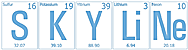# Chem 2B starts off with Review of 2A

Unit 8 Student Objectives: Stoichiometry-Part 1

• (8.01) I can use molar mass of a substance to convert between mass and the number of moles of a substance (from unit 5).
• (8.02) I can write a balanced chemical equation and relate the coefficients to the number of particles participating in the reaction.
• (8.03) I can calculate the number of moles of reactants consumed or products yielded in a reaction.
• (8.04) I can identify the limiting reactant or the reactant in excess in a given reaction.
• (8.05) I can determine the theoretical yield and calculate the percent yield by mass for a reaction.

Review of key aspects of S/GChem 2A, particularly for those who took 2A the first trimester.

• The seven diatomics
• Seven-UP
• BrIClHOF
• Naming rules.
• The table groups reviewed the difference between ionic and molecular compounds
• Molar Masses
• We practiced calculating molar masses.
• Some groups stumbled on aluminum sulfate.
• Mole/Mass Conversions
• The class finished practicing mole/mass conversions which we will whiteboard tomorrow.